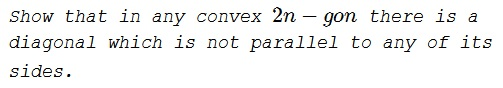# Diagonals in a Polygon Parallel to Sides

### Problem### Solution

The total number of diagonals in a convex $2n-gon$ is $\displaystyle\frac{1}{2}(2n)(2n - 3)=n(2n - 3).$

The diagonals which can be parallel to a fixed side must connect two vertices. The $2n - 2$ vertices not on the fixed side must include at least one vertex not on a diagonal parallel to the fixed side; hence there can be at most $\displaystyle\frac{1}{2}(2n-4)$ diagonals parallel to the fixed side, or altogether at most $\displaystyle 2n\cdot \frac{1}{2}\cdot(2n - 4) =n(2n - 4)$ diagonals which are parallel to some side. Of the remaining diagonals, at least $n(2n - 3) - n(2n - 4) = n$ are not parallel to any side.

### Acknowledgment

This is problem 67 from the Canadian Crux Mathematicorum (v1, 1975)/. The problem and the solution are by Viktor Linis.